##### Physics I For DummiesWhen an object moves in a circle, if you know the magnitude of the angular velocity, then you can use physics to calculate the tangential velocity of the object on the curve.

At any point on a circle, you can pick two special directions: The direction that points directly away from the center of the circle (along the radius) is called the radial direction, and the direction that’s perpendicular to this is called the tangential direction.

When an object moves in a circle, you can think of its instantaneous velocity (the velocity at a given point in time) at any particular point on the circle as an arrow drawn from that point and directed in the tangential direction. For this reason, this velocity is called the tangential velocity. The magnitude of the tangential velocity is the tangential speed, which is simply the speed of an object moving in a circle.

Given an angular velocity of magnitude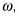the tangential velocity at any radius is of magnitude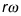The idea that the tangential velocity increases as the radius increases makes sense, because given a rotating wheel, you’d expect a point at radius r to be going faster than a point closer to the hub of the wheel.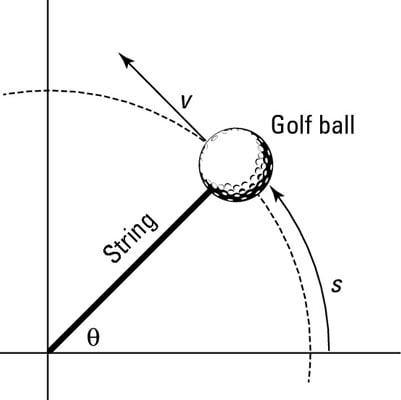A ball in circular motion has angular speed around the circle.

Take a look at the figure, which shows a ball tied to a string. The ball is whipping around with angular velocity of magnitude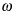You can easily find the magnitude of the ball’s velocity, v, if you measure the angles in radians. A circle has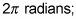the complete distance around a circle — its circumference — is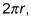where r is the circle’s radius. In general, therefore, you can connect an angle measured in radians with the distance you cover along the circle, s, like this: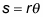where r is the radius of the circle. Now, you can say that v = s/t, where v is magnitude of the velocity, s is the distance, and t is time. You can substitute for s to get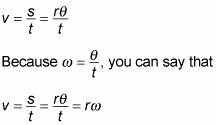In other words,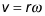Now you can find the magnitude of the velocity. For example, say that the wheels of a motorcycle are turning with an angular velocity of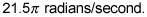If you can find the tangential velocity of any point on the outside edges of the wheels, you can find the motorcycle’s speed. Now assume that the radius of one of your motorcycle’s wheels is 40 centimeters. You know that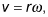so just plug in the numbers: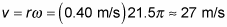Converting 27 meters/second to miles/hour gives you about 60 mph.